## 5 Functional description

06.423GPPTSVoice Activity Detection (VAD) for Half Rate Speech Traffic Channels

The purpose of this clause is to give the reader an understanding of the principles of operation of the VAD, whereas GSM 06.06  contains the fixed point computational description of the VAD. In the case of discrepancy between the two descriptions, the description in GSM 06.06  will prevail.

## 5.1 Overview and principles of operation

The function of the VAD is to distinguish between noise with speech present and noise without speech present. This is achieved by comparing the energy of a filtered version of the input signal with a threshold. The presence of speech is indicated whenever the threshold is exceeded.

The detection of speech in mobile environments is difficult due to the low speech/noise ratios which are encountered, particularly in moving vehicles. To increase the probability of detecting speech, the input signal is adaptively filtered (see clause 5.2.1) to reduce its noise content before the voice activity decision is made (see clause 5.2.7).

The frequency spectrum and level of the noise may vary within a given environment as well as between different environments. It is therefore necessary to adapt the input filter coefficients and energy threshold at regular intervals as described in clause 5.2.6.

## 5.2 Algorithm description

The block diagram of the VAD algorithm is shown in figure 1. The individual blocks are described in the following clauses. The global variables shown in the block diagram are described in table 1.

Table 1: Description of variables in figure 1

 Var Description acf The ACF vector which is calculated in the speech encoder (GSM 06.20 ). av0 Averaged ACF vector. av1 A previous value of av0. lags The open loop long term predictor lags for the four speech encoder subframes (GSM 06.20 ). ptch Boolean flag indicating the presence of a periodic signal component. pvad Energy in the current filtered signal frame. rav1 Autocorrelation vector obtained from av1. rc The first four unquantized reflection coefficients calculated in the speech encoder (GSM 06.20 ). rvad Autocorrelation vector of the adaptive filter predictor values. stat Boolean flag indicating that the frequency spectrum of the input signal is stationary. thvad Adaptive primary VAD threshold. tone Boolean flag indicating the presence of an information tone. vadflag Boolean VAD decision with hangover included. vvad Boolean VAD decision before hangover.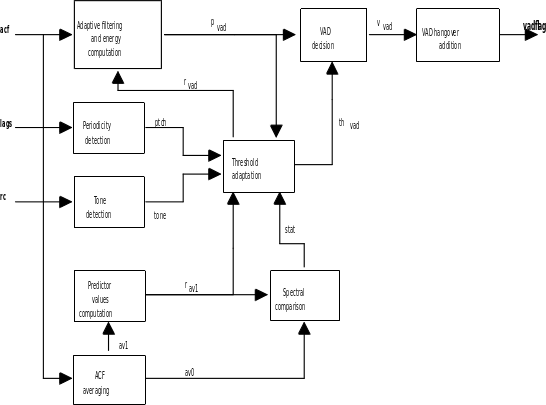Figure 1: Functional block diagram of the VAD

### 5.2.1 Adaptive filtering and energy computation

The energy in the current filtered signal frame (pvad) is computed as follows:

8

i=1

This corresponds to performing an 8th order block filtering on the filtered input samples to the speech encoder. This is explained in annex B.

### 5.2.2 ACF averaging

Spectral characteristics of the input signal have to be obtained using blocks that are larger than one 20 ms frame. This is done by averaging the ACF (autocorrelation function) vectors for several consecutive frames. The averaging is given by the following equations:

frames‑1

av0{n}[i] = SUM (acf{n-j}[i]) ; i = 0..8

j=0

av1{n}[i] = av0{n-frames}[i] ; i = 0..8

where (n) represents the current frame, (n‑1) represents the previous frame etc. The values of the constants and initial variable values are given in table 2.

Table 2: Constants and variables for ACF averaging

 Constant Value Variable Initial value frames 4 previous ACF’s, All set to 0 av0 & av1

### 5.2.3 Predictor values computation

The filter predictor values aav1 are obtained from the autocorrelation values av1 according to the equation: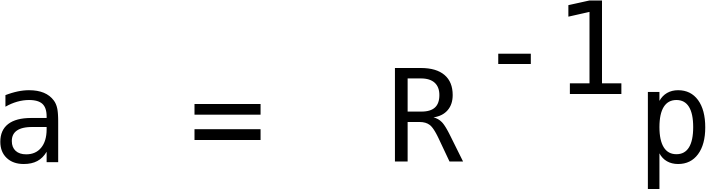where:

and: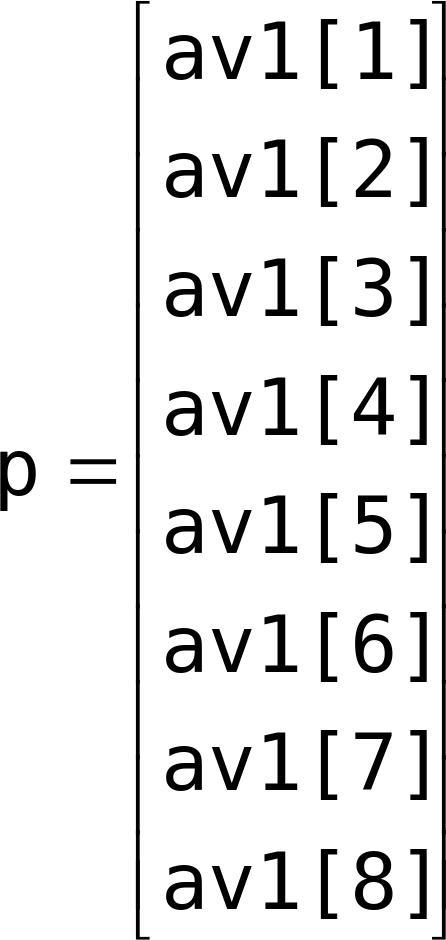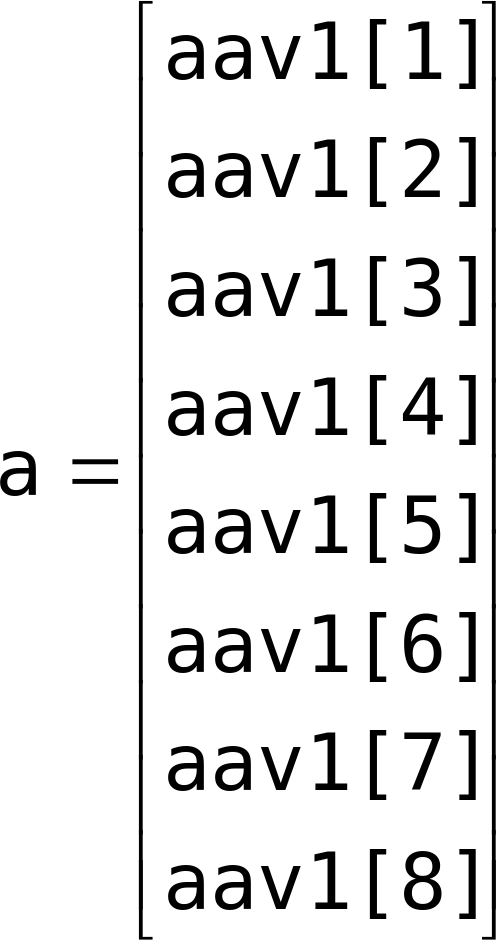aav1 = ‑1

av1 is used in preference to av0 as the latter may contain speech. The autocorrelated predictor values rav1 are then obtained:

8-i

rav1[i] = SUM (aav1[k]*aav1[k+i]) ; i = 0..8

k=0

### 5.2.4 Spectral comparison

The spectra represented by the autocorrelated predictor values rav1 and the averaged autocorrelation values av0 are compared using the distortion measure (dm), defined below. This measure is used to produce a Boolean value stat every 20 ms, as shown in the following equations:

8

dm = (rav1*av0 + 2*SUM (rav1[i]*av0[i]))/av0

i=1

difference = | dm – lastdm |

lastdm = dm

stat = (difference < thresh)

The values of the constants and initial variable values are given in table 3.

Table 3: Constants and variables for spectral comparison

 Constant Value Variable Initial value thresh 0,068 lastdm 0

### 5.2.5 Information tone detection

Information tones and noise can be classified by inspecting the short term prediction gain, information tones resulting in a higher prediction gain than noise. Tones can therefore be detected by comparing the prediction gain to a fixed threshold. By limiting the prediction gain calculation to a fourth order analysis, information signals consisting of one or two tones can be detected whilst minimizing the prediction gain for noise.

The prediction gain decision is implemented by comparing the normalized short term prediction error with the short term prediction error threshold (predth). This measure is used to produce a Boolean value, tone, every 20 ms. The signal is classified as a tone if the prediction error is less than predth. This is equivalent to a prediction gain threshold of 13,5 dB.

Vehicle noise can contain strong resonances at low frequencies, resulting in a high prediction gain. A further test is therefore made to determine the pole frequency of a second order analysis of the signal frame. The signal is classified as noise if the frequency of the pole is less than 385 Hz.

The algorithm for evaluating the Boolean tone flag is as follows:

tone = false

den = a*a

num = 4*a – a*a

if (num <= 0)

return

if ((a < 0) AND (num/den < freqth))

return

4

prederr = MULT (1 – rc[i]*rc[i])

i=1

if (prederr < predth)

tone = true

return

rc[1..4] are the first four unquantized reflection coefficients obtained from the speech encoder short term predictor. The coefficients a[0..2] are transversal filter coefficients calculated from rc[1..2]. The pole frequency calculation is described in annex C.

The values of the constants are given in table 4.

Table 4: Constants for information tone detection

 Constant Value freqth 0,0973 predth 0,0447

### 5.2.6 Threshold adaptation

A check is made every 20 ms to determine whether the adaptive primary VAD threshold, (thvad) should be changed. This adaptation is carried out according to the flow chart in figure 2. The values of the constants and initial variable values are given in table 5.

Adaptation of thvad takes place in two different situations:

In the first case, the decision threshold (thvad) is set to the lower limit for the adaptive threshold (plev) if the input signal frame energy (acf) is less than the energy threshold (pth). The autocorrelation vector of the adaptive filter predictor values (rvad) remains unchanged.

In the second case, thvad and rvad are adapted if there is a low probability that speech or information tones are present. This occurs when the following conditions are met:

a) the frequency spectrum of the input signal is stationary (clause 5.2.4);

b) the signal does not contain a periodic component (clause 5.2.9);

c) information tones are not present (clause 5.2.5).

The autocorrelation vector of the adaptive filter predictor values (rvad) is updated with the rav1 values. The step size by which thvad is adapted is not constant but a proportion of the current value and its rate of increase or decrease is determined by constants inc and dec respectively.

The adaptation begins by experimentally multiplying thvad by a factor of (1‑1/dec). If thvad is now higher than or equal to pvad times, the steady state adaptive threshold constant (fac), then thvad needed to be decreased and it is left at this new lower level. If, on the other hand, thvad is less than pvad times fac, then it either needs to be increased or kept constant. In this case, it is multiplied by a factor of (1+1/inc) or set to pvad times fac whichever yields the lower value. thvad is never allowed to be greater than pvad+upper adaptive threshold limit (margin).

Table 5: Constants and variables threshold adaptation

 Constant Value Variable Initial value pth 210000 margin 112000000 plev 560000 adaptcount 0 fac 2,55 thvad 1400000 adp 8 rvad 6 inc 16 rvad to All 0 dec 32 rvad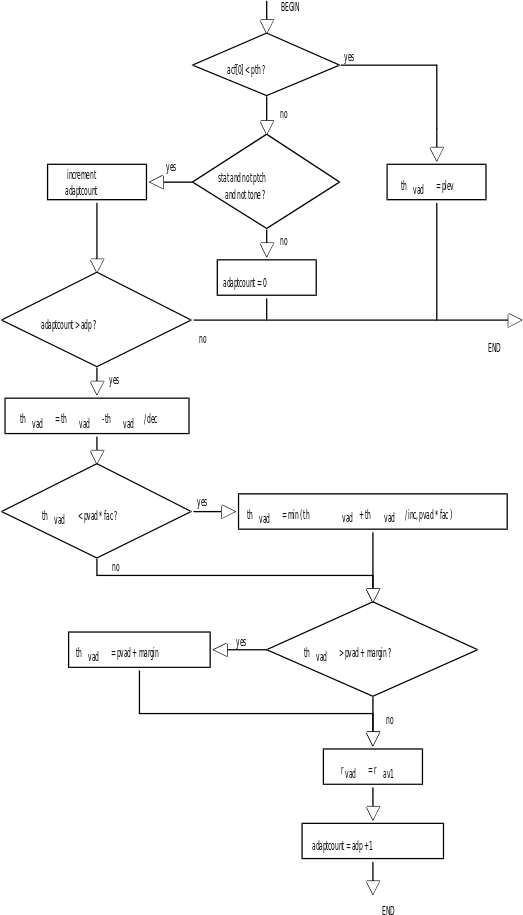Figure 2: Flow diagram for threshold adaptation

### 5.2.7 VAD decision

Prior to hangover the Boolean VAD decision is defined as:

VAD hangover is only added to bursts of speech greater than or equal to burstcount blocks. The Boolean variable vadflag indicates the decision of the VAD with hangover included. The values of the constants and initial variable values are given in table 6. The hangover algorithm is as follows:

increment(burstcount)

else

burstcount = 0

if (burstcount >= burstconst)

{

hangcount = hangconst

burstcount = burstconst

}

vadflag = (vvad OR (hangcount >= 0))

if (hangcount >= 0)

decrement(hangcount)

Table 6: Constants and variables for VAD hangover addition

 Constant Value Variable Initial value burstconst 3 burstcount 0 hangconst 5 hangcount ‑1

### 5.2.9 Periodicity detection

thvad and rvad are updated when the frequency spectrum of the input signal is stationary. However, vowel sounds also have a stationary frequency spectrum. The Boolean variable ptch indicates the presence of a periodic signal component and prevents adaptation of thvad and rvad. ptch is updated every 20 ms and is true when periodicity (a vowel sound) is detected. The periodicity detector identifies the vowel sounds by comparing consecutive Long Term Predictor (LTP) lag values lags[1..4] which are obtained every sub frame from the speech codec defined in GSM 06.20 . Cases in which one lag value is a factor of the other are catered for. However, consecutive lags with a ratio which is either non‑integer or greater than 3 are not classified as periodic.

lagcount = 0

for ( j=1; j<=4; j++ )

{

smallag=maximum(lags[j],lags[j‑1])

for ( i=1; i<=3; i++ )

if (smallag >= minimum(lags[i], lags[i‑1]))

smallag = smallag – minimum(lags[i], lags[i‑1])

if (minimum(smallag,minimum(lags[j],lags[j‑1])-smallag)<lthresh)

increment(lagcount)

}

veryoldlagcount = oldlagcount

oldlagcount = lagcount

ptch = (oldlagcount + veryoldlagcount >= nthresh)

The values of constants and initial values are given in table 7. lags = lags of the previous frame.

ptch is calculated after the VAD decision and when the current LTP lag values lags[1..4] are available. This reduces the delay of the VAD decision.

Table 7: Constants and variables for periodicity detection

 Constant Value Variable Initial value lthresh 2 ptch 1 nthresh 7 oldlagcount 0 veryoldlagcount 0 lags 21